# Difference between revisions of "Beeman's algorithm"

Beeman's algorithm  is is a method for numerically integrating ordinary differential equations, generally position and velocity, which is closely related to Verlet integration.

In its standard form, it produces the same trajectories as the Verlet algorithm, but the velocities are more accurate: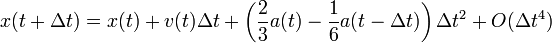$x(t+\Delta t) = x(t) + v(t) \Delta t + \left(\frac{2}{3}a(t) - \frac{1}{6} a(t - \Delta t) \right)\Delta t^2 + O( \Delta t^4)$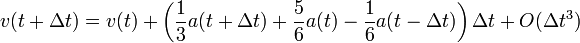$v(t + \Delta t) = v(t) + \left(\frac{1}{3}a(t + \Delta t) + \frac{5}{6}a(t) - \frac{1}{6}a(t - \Delta t) \right) \Delta t + O(\Delta t^3)$

where x is the position, v is the velocity, a is the acceleration, t is time, and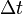$\Delta t$ is the time-step.

A predictor-corrector variant is useful when the forces are velocity-dependent:$x(t+\Delta t) = x(t) + v(t) \Delta t + \frac{2}{3}a(t) \Delta t^2 - \frac{1}{6} a(t - \Delta t) \Delta t^2 + O( \Delta t^4).$

The velocities at time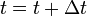$t =t + \Delta t$ are then calculated from the positions.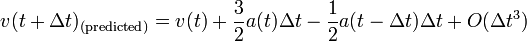$v(t + \Delta t)_{(\mathrm{predicted})} = v(t) + \frac{3}{2}a(t) \Delta t - \frac{1}{2}a(t - \Delta t) \Delta t + O( \Delta t^3)$

The accelerations at time$t =t + \Delta t$ are then calculated from the positions and predicted velocities.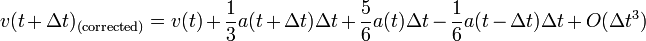$v(t + \Delta t)_{(\mathrm{corrected})} = v(t) + \frac{1}{3}a(t + \Delta t) \Delta t + \frac{5}{6}a(t) \Delta t - \frac{1}{6}a(t - \Delta t) \Delta t + O( \Delta t^3)$